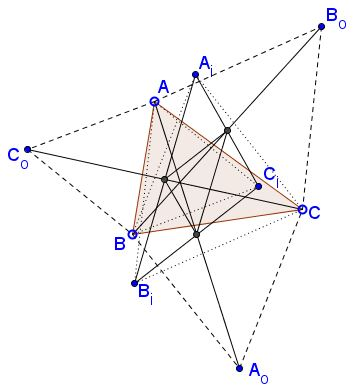# Midpoint Reciprocity in Napoleon's Configuration

### Problem

Let $ABC_{o},$ $BCA_{o},$ $CAB_{o}$ be the outer, $ABC_{i},$ $BCA_{i},$ $CAB_{i}$ the inner Napoleon's triangles.Then the midpoints $AA_{o}$ and $B_{i}C_{i}$ coincide $(A_{m})$ as are the midpoints of $BB_{o},$ $C_{i}A_{i}$ $(B_{m})$ and $CC_{o},$ $A_{i}B_{i}$ $(C_{m}).$ A similar assertion holds with the exchange of the indices $_{o}$ and $_{i}$ throughout.

### Proof

We'll use complex numbers. Let $\omega = \cos 60^{\circ}+i\sin 60^{\circ}$ be a counterclockwise rotation through $60^{\circ}.$ Treating points as complex numbers,

\begin{align} A_{o}&=C+(B-C)\omega\\ B_{i}&=C+(A-C)\omega\\ C_{i}&=A+(B-A)\omega. \end{align}

so that

$\displaystyle\frac{A+A_{o}}{2}=\frac{(A+C)+(B-C)\omega}{2}.$

Also,

$\displaystyle\frac{B_{i}+C_{i}}{2}=\frac{(A+C)+(B-C)\omega}{2}$

and the proof is complete as other midpoints are treated similarly

### Acknowledgment

The statement has been posted by Dao Thanh Oai (Vietnam) at the CutTheKnotMath facebook page.### Napoleon's Theorem